Question

A chemist titrates 90.0 mL of a 0.5705 M carbonic acid (H,CO,) solution with 0.6274 M...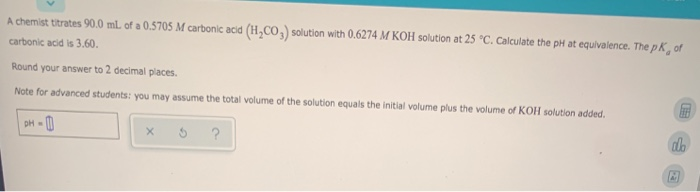A chemist titrates 90.0 mL of a 0.5705 M carbonic acid (H,CO,) solution with 0.6274 M KOH solution at 25 °C. Calculate the pH at equivalence. The pk of carbonic acid is 3.60. Round your answer to 2 decimal places. Note for advanced students: you may assume the total volume of the solution equals the initial volume plus the volume of KOH solution added. DH-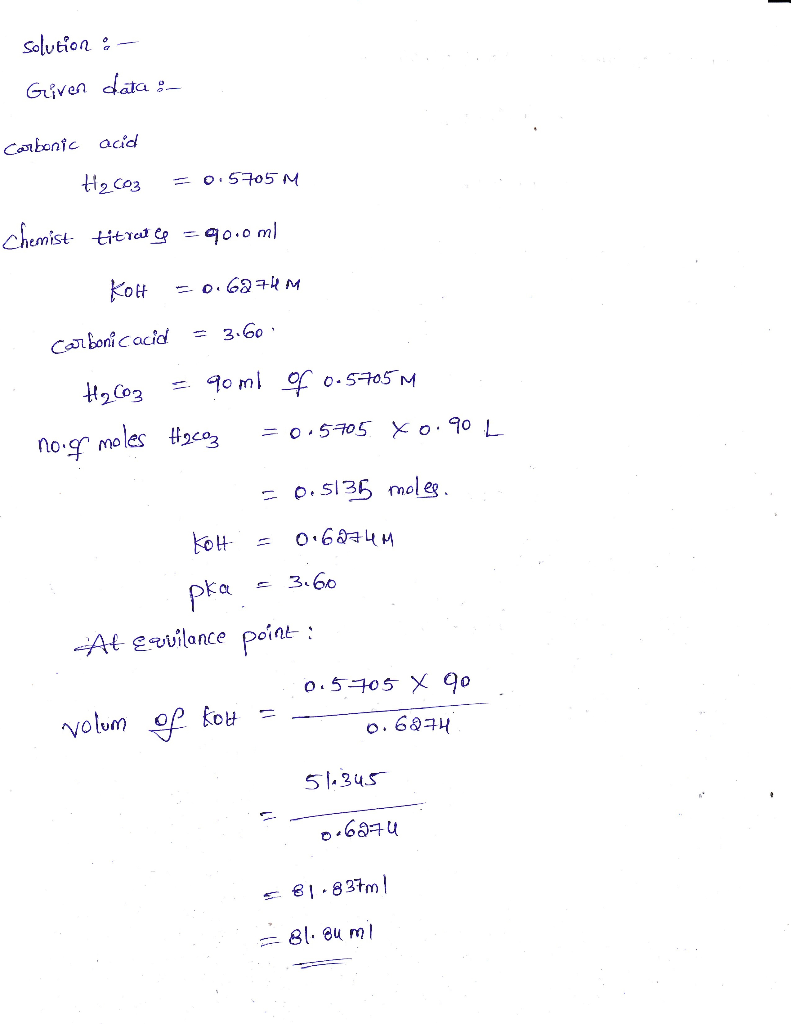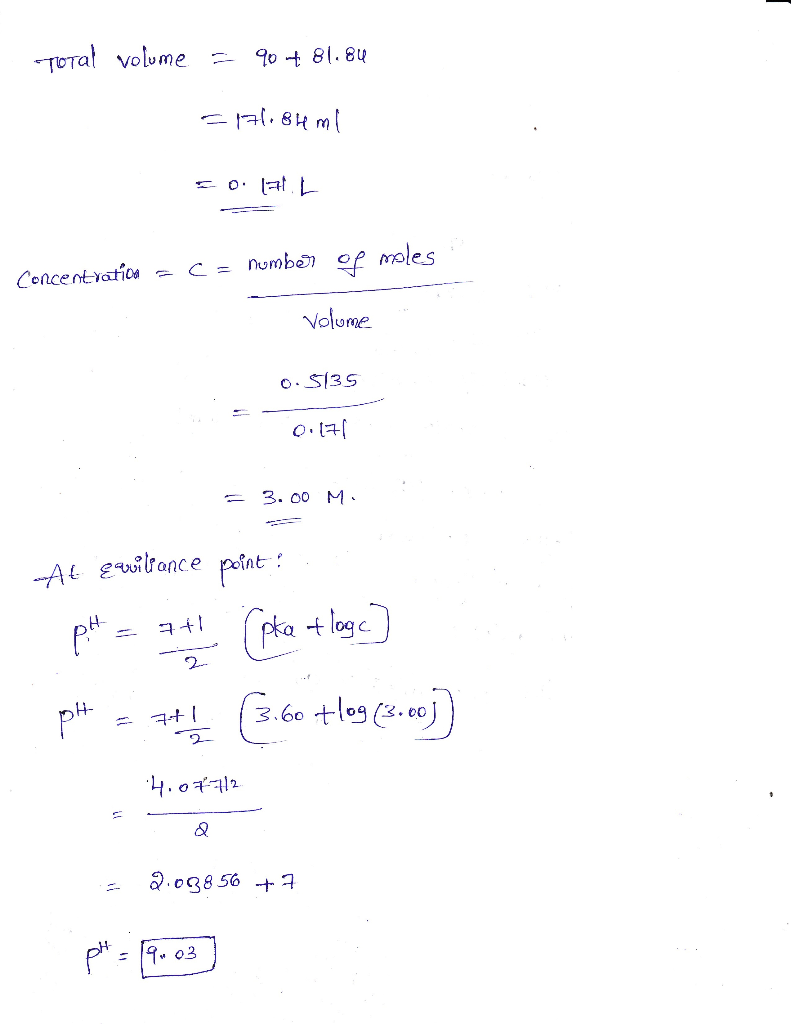Earn Coins

Coins can be redeemed for fabulous gifts.

Similar Homework Help Questions
• A chemist titrates 130.0 mL of a 0.5266 M carbonic acid (H.CO) solution with 0.5751 M...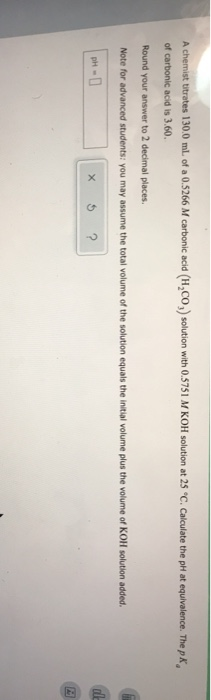A chemist titrates 130.0 mL of a 0.5266 M carbonic acid (H.CO) solution with 0.5751 M KOH solution at 25 °C. Calculate the pH at equivalence. The pk of carbonic acid is 3.60. Round your answer to 2 decimal places. Note for advanced students: you may assume the total volume of the solution equals the initial volume plus the volume of KOH solution added. en de ?

• A chemist titrates 160.0 mL of a 0.3337 M carbonic acid (H2CO3) solution with 0.4095 M...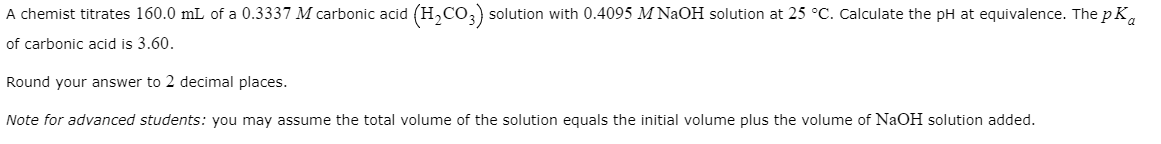A chemist titrates 160.0 mL of a 0.3337 M carbonic acid (H2CO3) solution with 0.4095 M NaOH solution at 25 °C. Calculate the pH at equivalence. The pK, of carbonic acid is 3.60. Round your answer to 2 decimal places. Note for advanced students: you may assume the total volume of the solution equals the initial volume plus the volume of NaOH solution added.

• Calculating the pH at equi.. A chemist titrates 70.0 ml. of a 04557 M carbonic acid...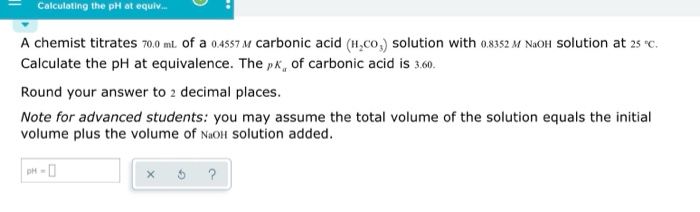Calculating the pH at equi.. A chemist titrates 70.0 ml. of a 04557 M carbonic acid (H,co,) solution with o8352 M NaOlH Solution at 2s c Calculate the pH at equivalence. The pk, of carbonic acid is 3.60 Round your answer to 2 decimal places. Note for advanced students: you may assume the total volume of the solution equals the initial volume plus the volume of NaoH Solution added. рH - D

• A chemist titrates 190.0 mL of a 0.4425 M cyanic acid (HCNO) solution with 0.3359 M...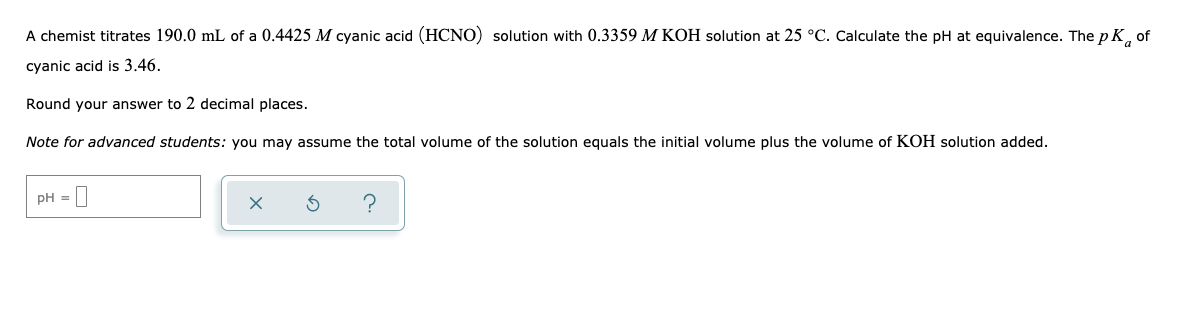A chemist titrates 190.0 mL of a 0.4425 M cyanic acid (HCNO) solution with 0.3359 M KOH solution at 25 °C. Calculate the pH at equivalence. The pK, of cyanic acid is 3.46. Round your answer to 2 decimal places. Note for advanced students: you may assume the total volume of the solution equals the initial volume plus the volume of KOH solution added. pH = D x 6 ? |

• A chemist titrates 190.0 mL of a 0.4427 Macetic acid (HCH,CO, solution with 0.2382 M NaOH...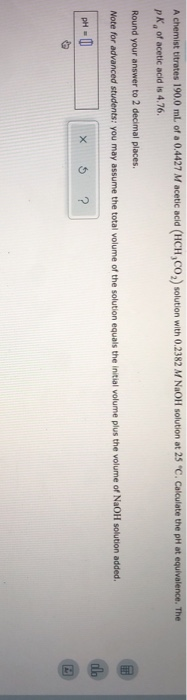A chemist titrates 190.0 mL of a 0.4427 Macetic acid (HCH,CO, solution with 0.2382 M NaOH solution at 25 "C. Calculate the pH at equivalence. The pk of acetic acid is 4.76. Round your answer to 2 decimal places. Note for advanced students: you may assume the total volume of the solution equals the initial volume plus the volume of NaOH solution added. pH = 0 x 5 ?

• - Calculating the pH at equivalence of a titration A chemist titrates 180.0 ml of a...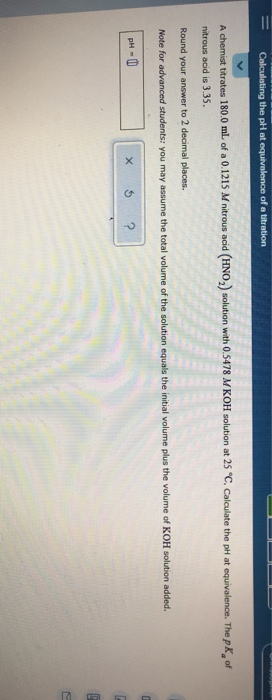- Calculating the pH at equivalence of a titration A chemist titrates 180.0 ml of a 0.1215 M nitrous acid (HNO) solution with 0.5478 M KOH solution at 25 "C. Calculate the pH at equivalence. The pk of nitrous acid is 3.35. Round your answer to 2 decimal places. Note for advanced students: you may assume the total volume of the solution equals the initial volume plus the volume of KOH solution added. PHU X 5 ?

• A chemist titrates 200.0 mL of a 0.7681 M hydrocyanic acid (HCN) solution with 0.5271 M...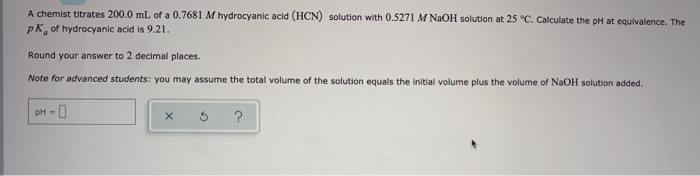A chemist titrates 200.0 mL of a 0.7681 M hydrocyanic acid (HCN) solution with 0.5271 M NaOH solution at 25 °C. Calculate the pH at equivalence. The pk of hydrocyanic acid is 9.21. Round your answer to 2 decimal places. Note for advanced students: you may assume the total volume of the solution equals the initial volume plus the volume of NaOH solution added. pH- х ?

• A chemist titrates 100.0 ml of a 0,6612 M hydrocyanic acid (HCN) solution with 0.8512 M NaOH solution at 25 c. Calc...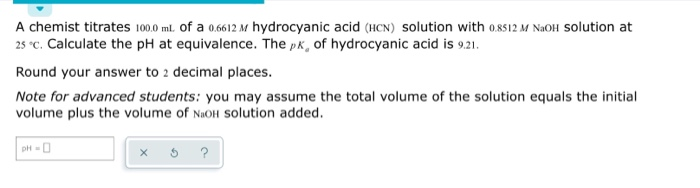A chemist titrates 100.0 ml of a 0,6612 M hydrocyanic acid (HCN) solution with 0.8512 M NaOH solution at 25 c. Calculate the pH at equivalence. The pk, of hydrocyanic acid is 9.21. Round your answer to 2 decimal places. Note for advanced students: you may assume the total volume of the solution equals the initial volume plus the volume of N.OH solution added. pH- x 5 ?

• please show clear explanation Thank you! A chemist titrates 220.0 mL of a 0.4581 M hydrocyanic...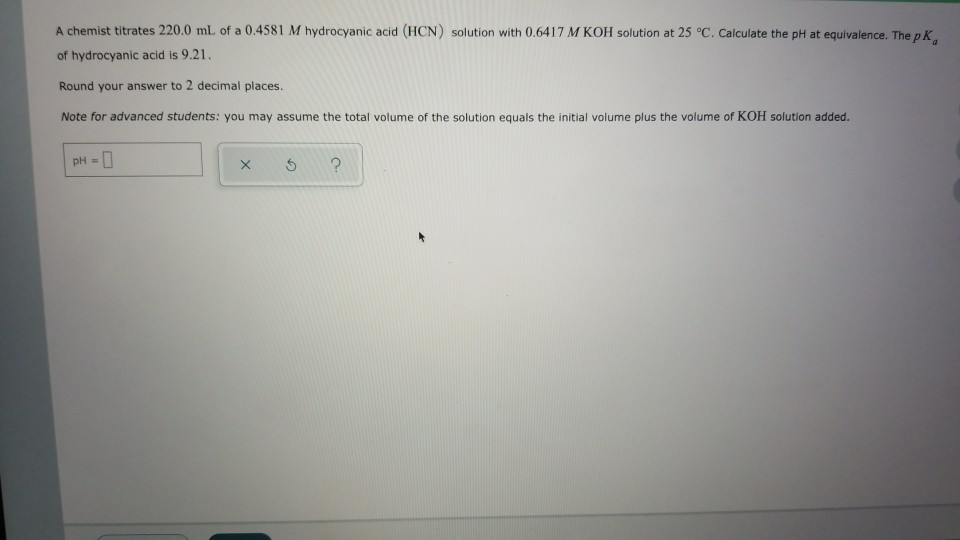please show clear explanation Thank you! A chemist titrates 220.0 mL of a 0.4581 M hydrocyanic acid (HCN) solution with 0.6417 M KOH solution at 25 °C. Calculate the pH at equivalence. The pk of hydrocyanic acid is 9.21. Round your answer to 2 decimal places. Note for advanced students: you may assume the total volume of the solution equals the initial volume plus the volume of KOH solution added. pH = 1 X 5 ?

• An analytical chemist is titrating 183.2 mL of a 1.000 M solution of butanoic acid (HC,H,CO,...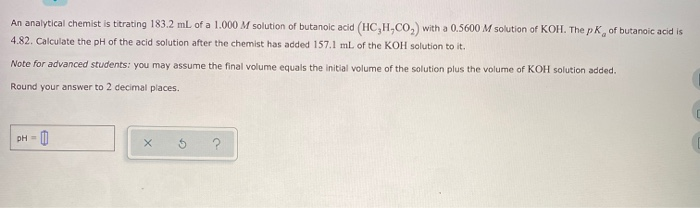An analytical chemist is titrating 183.2 mL of a 1.000 M solution of butanoic acid (HC,H,CO, with a 0.5600 M solution of KOH. The pk of butanoic acid is 4.82. Calculate the pH of the acid solution after the chemist has added 157.1 mL of the KOH solution to it. Note for advanced students: you may assume the final volume equals the initial volume of the solution plus the volume of KOH solution added. Round your answer to 2 decimal...